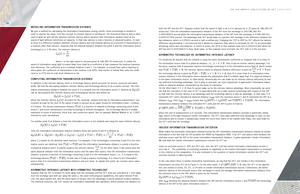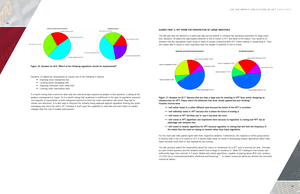x

#### A PHP Error was encountered

Severity: Notice

Message: Undefined variable: content_category

Filename: user/transcript.php

Line Number: 106

#### A PHP Error was encountered

Severity: Warning

Message: Invalid argument supplied for foreach()

Filename: user/transcript.php

Line Number: 106On The Impact and Future of HFT: White Paper

Total Views  :   1551
Total Likes  :  0
Total Shares  :  0

Presentation Slides

1) ON THE IMPACT AND FUTURE OF HFT W H I T E PA P E R Khaldoun Khashanah Ionut Florescu Steve Yang Financial Engineering Division School of Systems and Enterprises Stevens Institute of Technology May 10, 2014 This work was supported by the Investor Responsibility Research Center Institute.

10) ON THE IMPACT AND FUTURE OF HFT WHITE PAPER 16 MODELING INFORMATION TRANSMISSION DISTANCE We give a method for calculating the information transmission zoning cutoffs. Some terminology is needed in order to express the ideas. First the concept of intrinsic latency is introduced. The theoretical latency that is often used is what we will call the absolute intrinsic latency, which assumes that information packets travel at the speed of light without restrictions on capacity. In that case latency is only a function of physical location. In reality there is a difference between absolute intrinsic latency and real network latency as a function of transmission in a medium other than vacuum. Suppose that the physical distance between the point A and the information source (exchange) is miles. The intrinsic latency is both the SIP and the HFT. Suppose further that the speed of light is as it is in vacuum ( given at 186,282.40 mi/second. Then the information transmission distance of the SIP from the exchange is 100/186,282.40= 0.000536819 second while the information transmission distance of the SIP from the exchange is 2/186,282.40= 0.0000107364 second. The advantage that HFT would have on a generic SIP message is the difference between the two distances, which is 0.00052 second or half a millisecond. Changing the TCP to UDP for HFT affects the latency difference approximately 1 microsecond but it allows for maximum flow, which is necessary for HFT to accommodate streaming orders and cancellations. In terms of zones, the HFTs in this example have |z1|=0.0000107364 while the SIP has |z2|=0.000536819 in their ideal cases. In this example since |z1|&lt;|z2|, the HFT unit is in the red zone. ASYMMETRIC TECHNOLOGY VS. ASYMMETRIC INTRINSIC LATENCY where c is the light speed in vacuum given at 186,282.40 mi/second. In reality the speed of information using light is scaled down from ideal by a coefficient that measures the medium transmission efficiency. The coefficient is a constant between 0 and 1. For example, in normal fiber optics traveling in silica glass, the coefficient of transmission of the medium is =69%. New reports of hollow fiber raise the coefficient to 97% but for only short distances so far. COMPUTING INFORMATION TRANSMISSION DISTANCE In addition to the intrinsic latency, there is technology latency, which accounts for servers, protocols and bandwidth. We find the decoupling between intrinsic latency and technology latency to be a useful concept. The information transmission distance between the point A in a network and the information source E denoted by can be decomposed into intrinsic latency and technological latency and written as where the intrinsic latency accounts for the universal &ldquo;physics time-tax on information transmission&rdquo; represented at least by the limit of the speed of light in vacuum as an upper bound for information travel&mdash;courtesy of Einstein. The excess transmission distance is a function of network technology connecting point A and source E and server transmission-receiving technology to achieve throughput. The technology latency is usually expressed in terms of protocols, block size, and connection speed. See for example Mathew Mathis et. al. (1997) formula for such calculations . For another point B at distance y from the information source in the network and using the same intrinsic latency, . stands for the absolute value function. For example, if the technologies connecting A and B to the infor- mation source are identical, then the information source than B in physical distance, i.e., . Then A has an inrinsic latency advantage. Furthermore, suppose that B possesses superior technology connecting it to the information source than A, which means The intrinsic latency based on physical distance difference is given by that and If then B is closer than A in information transthe technology latency is given by mission distance to the information source despite the assumption that B is farther away than A in physical distance to the same information source. In other words, theoretically one can make up for intrinsic latency deficiency by having sufficient technology advantage. This is why, in principle, we reject arguments of asymmetric information based solely on physical distance represented by the issue of colocation. On the other hand, if then B cannot make up for the intrinsic latency advantage. More importantly, we point out that the colocation in the case of HFT is associated with up-to-date superior technology with respect to the SIP so that both the intrinsic latency is an advantage and the technology latency is also an advantage. In that case, If A represents HFT units colocated at physical distance from the exclusive source E and B represents the SIP at a distance , then means that and . The information transmission distance between the colocated HFT units and the SIP is given in total by where the unit of measurement is in seconds. This information transmission distance provides a systematic advantage, which is the high frequency insider information. The HFT units have sufficient time advantage to react on this information and to convert it dynamically, inside the micro-time frame of the market order flows, into cash flows for the HFT units and the exchange. INSIDER INFORMATION TRANSMISSION CRITERION Then the information transmission distance between those two points A and B is defined as where For simplicity we assume that the network is using the same transmission coefficient . Suppose that A is closer to and the information transmission distance is purely a function of physical distance and it is exactly equal to the intrinsic latency On the other hand, if two points have the same physical distance from the information source and the same fiber optics, then the information transmission distance is purely a function of technology connecting A and B to the information source, i.e., it is equal to the technology latency In that case if A has a superior technology, A is closer to the information source than B in information transmission distance and vice versa. To explain this point, we consider some cases subsequently. ASYMMETRIC INTRINSIC LATENCY WITH SYMMETRIC TECHNOLOGY Suppose that the SIP is located 50 miles away from the exchange and the HFT units are colocated at 1 mile away from the exchange and both are using the same x, the exact technological capabilities, the same Internet Protocols, the same packet size, and the same types of servers, then the advantage is purely physical location distance. The identical protocols, say TCP, require an information handshake and validation, which doubles the distance for What makes the actionable information obtained below the SIP information transmission distance classify as insider information is the fact that the SIP provides the NBBO by Regulation NMS. If an HFT unit places itself between the exclusive source and the SIP in the sense information transmission distance, it gains systematic insider information. We now state the insider information transmission criterion for HFT as: Given an exclusive source E, SIP, and HFT unit, then the HFT unit has insider information transmission access if and only if . The possibility of converting positively or negatively on the insider information transmission is irrelevant to the criterion or the designation. It is also irrelevant to the question of insider information whether the HFT unit provides liquidity or takes liquidity. In the case where there is insider information transmission, we say that the HFT unit resides in the information transmission red zone (Z1) as in Figure 5. On the other hand, if , then the HFT or the algorithmic unit resides in zones, Z2, Z3 , or Z4 with no insider information transmission access. Furthermore, we define systemic latency of the exclusive source (the exchange) to mean the average information transmission distance from the exclusive source to the SIP, which is given by the formula with denoting the physical distance between the SIP and the information source E and latency of the SIP to the same information source E. the technology 17